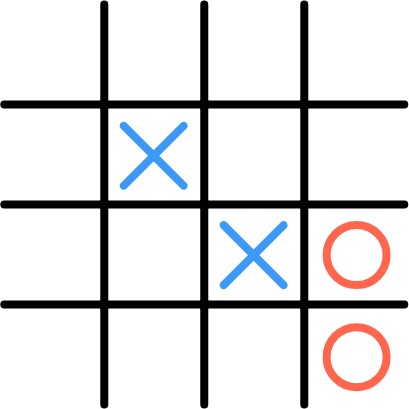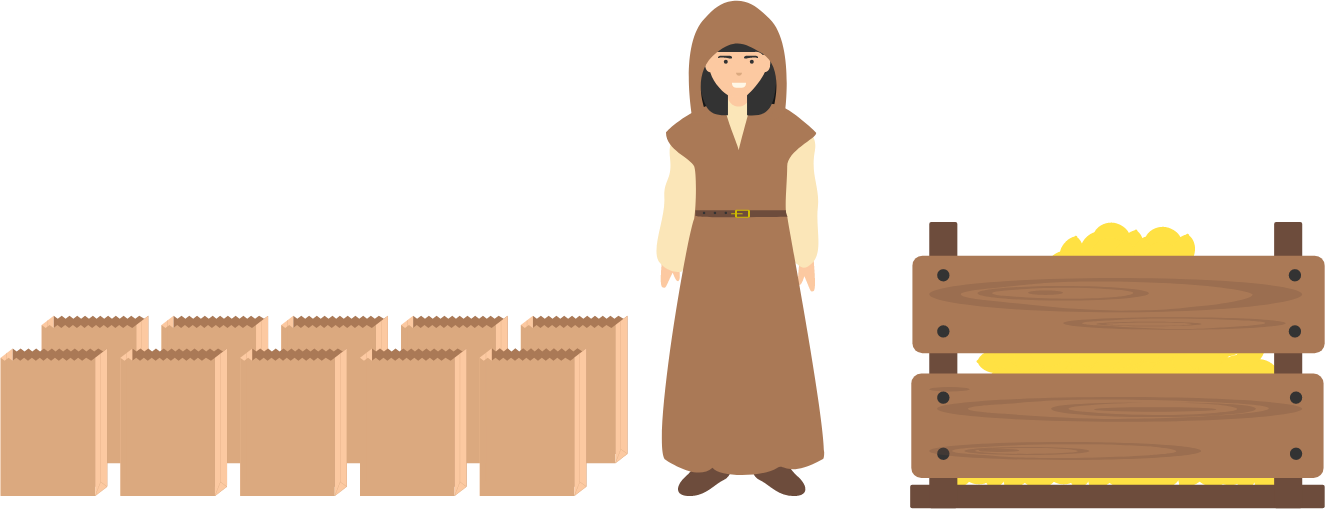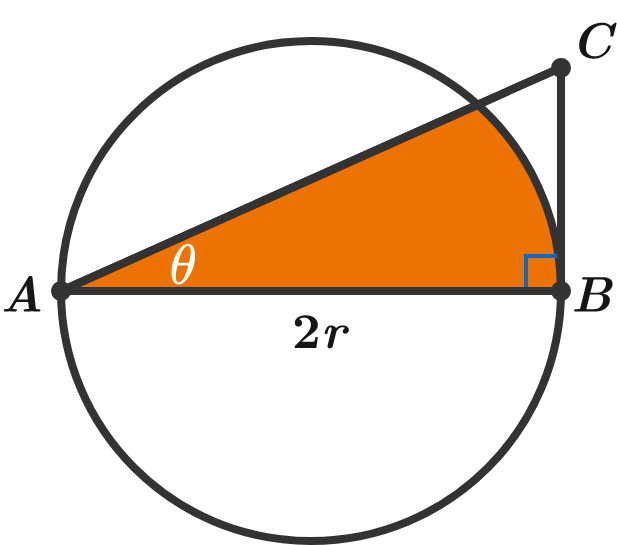# Problems of the Week

Contribute a problem

# 2018-12-17 IntermediateIn this example, Xavier begins by placing 2 Xs along a diagonal. Then, Olivia plays two Os along the right column.

Xavier and Olivia play a variation of tic-tac-toe on a 4 $\times$ 4 board in which they alternate placing 2 letters on each turn. The first player to get 4 of their letters in a horizontal, vertical, or diagonal row is the winner.

If Xavier goes first and both players play optimally, who will win?

Note: The image is an example of play, and does not necessarily represent optimal play for either player.A mysterious stranger approaches you and hands you 1023 lemons and 10 bags. The stranger says, "Arrange these lemons in these bags however you like, but soon you will be required to give a specific number of lemons (by handing over some bag or bags), and you cannot transfer the lemons between bags once you have arranged them all. Good luck!" Then, she walks away.

After you finish arranging the lemons, a friend suddenly approaches you and frantically asks, "Quick! There's no time to explain! I need exactly $n$ lemons!"

Can you fulfill your friend's request for any positive integer $n$ less than or equal to 1023?

$\large A = 1 + \frac{1}{ 1 + \frac{ 1}{ 1 + \frac{ 1} { \ddots } } } \qquad \text{and}\qquad B = 2 + \frac{1}{ 2 + \frac{ 1}{ 2 + \frac{ 1} { \ddots } } }$

Given the two infinite continued fractions $A$ and $B$ above, which is larger, $2A$ or $B?$

$AB$ is the diameter of the circle.

What is the orange area in terms of $r$ and $\theta ?$\begin{cases} \begin{aligned} x\ + \ y\ +\ z \ & =12 \\ x^2+y^2+z^2 & =12 \\ x^3+y^3+z^3 & =12 \end{aligned} \end{cases}

If $x,y,$ and $z$ satisfy the system of equations above, what is the value of $x^4+y^4+z^4?$

×